# The estimated velocity of open loop does not match the observed velocity of back electromotive force

cancel
Showing results for
Did you mean:

## The estimated velocity of open loop does not match the observed velocity of back electromotive force

227 ViewsContributor II

I ported the S32K144 demo program MCSPTE1AK144_PMSM_FOC_1Sh to MPC5744P and found that the electrical angular velocity estimated by the CalcOpenLoop()  was twice the actual electrical angular velocity and twice the electrical angular velocity observed by the BEMF observer(pospeSensorless. WRotEl).

The estimated electrical angular velocity by the CalcOpenLoop() is the same as the electrical angular velocity which I set.

this is the electrical angular velocity estimated by the CalcOpenLoop():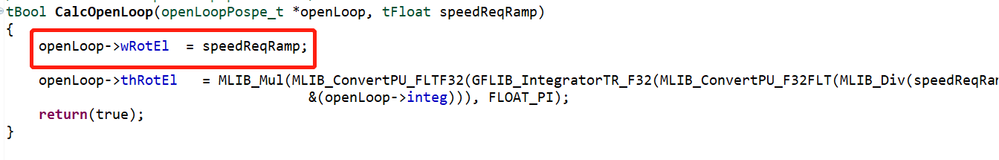Which leads to the unknown how to cut into the closed loop。

But when I halve the frequency of AMCLIB_TrackObsrv_FLT, the pospeSensorless. WRotEl is equal to pospeControl. WRotEl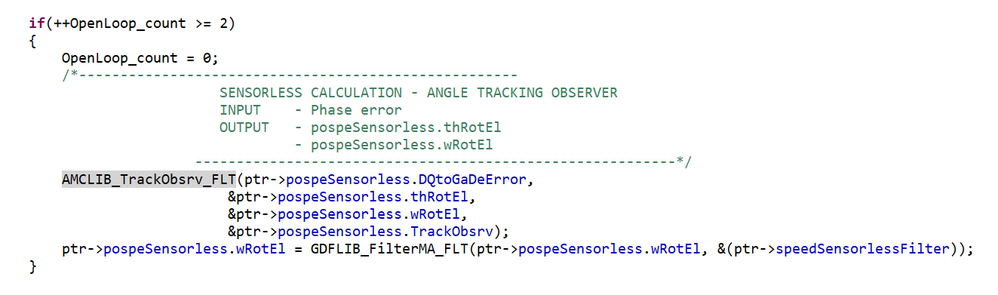3 Replies
201 ViewsNXP Employee

Hello,

CalcOpenLoop() and AMCLIB_TrackObsrv_FLT() must be called periodically with the same sampling frequency. In the case of MCSPTE1AK144_PMSM_FOC_1Sh, these functions are executed in the ADC interrupt service routine every 150us. This value (150us) appears also in MCAT as parameter sampling time. You have to make sure that this time is set properly according to your application. If this time is set wrong then also your position will be wrong, because parameters of the integrators are derived from this sampling time.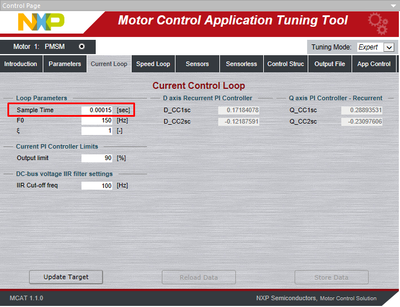Another parameter I would definitely check is pole pairs number pp in MCAT. This might also cause differences. Or do you use the same motor as MCSPTE1AK144 has ?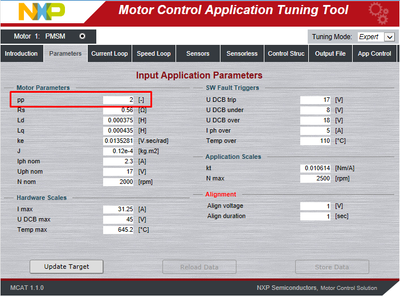Regards,

Marek

192 ViewsContributor II

CalcOpenLoop() and AMCLIB_TrackObsrv_FLT() have the same frequency in my program.
I have now solved this problem by doubling SCALAR_INTEG_GAIN so that the estimated speed in CalcOpenLoop() is the same as that observed by the observer.
But it's not clear to me why that would solve the problem, that I don't understand the rationale behind this integral, right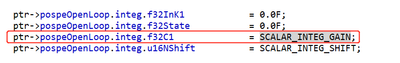I have another question I would like your help with, namely the definition of I_MAX and U_DCB_MAX, which I read in another post refers to the ratio between the actual voltage/current values and the ADC measurements.
So in my program, I need to multiply the ADC value by 800 to get the actual voltage value, so my U_DCB_MAX is 800, is that right?
My current value is the value obtained by the ADC minus the 2.5V offset voltage, and then multiplied by 100, so my I_MAX is 100, is that right?

Best regards，

Edison_Li

215 ViewsNXP Employee

Hello,

I have asked motor control expert for S32K1 to have a look at this.

Best regards,

Peter